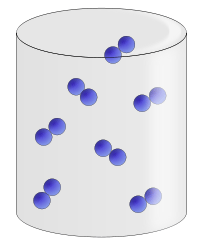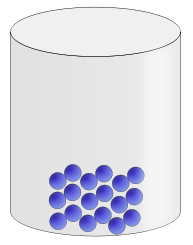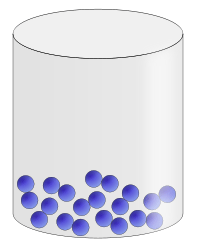Chemistry µGCSE:
States (GCSE)

10 minutes maximum! (can you do it in 5?)

Q1-3. The diagram shows particles of a substance in three states of matter.X Y Z
1. Which of the following statements is true about diagrams X, Y and Z?
• A.   ‘X’ shows a gas and  ‘Y’ shows a liquid
• B.   ‘X’ shows a gas and  ‘Z’ shows a solid
• C.   ‘Z’ shows a gas and  ‘X’ shows a liquid
• D.   ‘Z’ shows a liquid and  ‘Y’ shows a solid

2. Which of these diagrams shows the particles with the most and the least energy?

 Most energy Least energy A X Z B X Y C Y X D Y Z

3. Which change occurs during freezing?

• A.   Z to Y
• B.   X to Y
• C.   Y to Z
• D.   X to Z

4. The particles vibrate about a fixed position in a ….

• A.   gas
• B.   liquid
• C.   solid
• D.   solution

5. In which substance are the particles close together and slowly moving past each other?

• A. steam
• B. water
• C. ice
• D. oxygen
6. The particles in molten wax are arranged differently compared to solid wax. The particles in molten wax are …….
• A. closer together and moving faster.
• B. closer together and moving slower.
• C. further apart and moving faster.
• D. further apart and moving slower.

Q7-9: These questions concern changes of state.

What is the name of the interconversion when ……

7. ...molten iron changes to solid iron.
8. ...steam changes to water.
9. ...ice changes to water.

10. Substance P has a melting point of -35°C and a boiling point of 86°C.

Substance Q melts at 54°C and boils at 205°C.

At room temperature (25°C) the states of substances P and Q are:

 P Q A solid solid B solid liquid C liquid solid D gas liquid